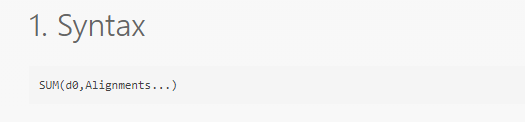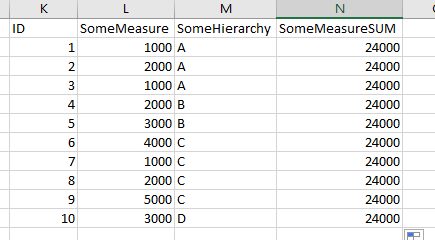# Using alignments in data cube calculations

Hi All,

I’m wondering how to use alignments in formulas in the data cube, calculated element transformation…As illustration this is what I want to end up with:

But when adding a calculated element like this: return SUM(\$SomeMeasure\$,\$SomeHierarchy\$)I need to keep the ID in the result (and I have a bunch of other fields in the data cube also, so I haven’t been able to get the result I need with the aggregate transform.)

This works excellent when calculated in the metric set, but I want to re-use this calculation across many metric sets, hence why I want the calculation in the data cube.

What I’m I missing here?

@ole.christian.valstad hi, hierarchy alignments is a formula parameter and therefore will only work for formulas, it is not supported in calculated elements. As you mentioned, it works in formulas in the metric set level, and that’s the way to go to get the proper aligned results.

Hi @jay.gong
Thanks for your answer. I suspected as much, but wasn’t sure since I couldn’t find anything specific about it in the documentation.

If this was possible I think it would take the data cube functionality to the next level, but I see that this might be quite complicated to implement since the alignments refer to hierarchies and hierarchies do not exist until the ‘process result’ step?

If I understood correctly your case, you can try to calculate this values directly in a query and you can further use this cube for other scenarios. As an example, you will have one data flow into the cube with this calculation and another flow for the other columns/values. In order to map these data flows you can use unions, joins etc.

1 Like

Yes indeed thanks for your input.

And that is how I ended up solving it. Creating a separate cube holding this calculation, and then that can be easily joined in to other cubes.

One drawback with this is that we do have a quite complex data architecture, and therefore I try to limit the number of cubes as much as possible - because I don’t want to end up with thousands of cubesAlso the cube where I want to have this is - use only other cubes as source so cannot use SQL.

But again I ended up just having a query going directly to the source, creating a data cube for this purpose only, to be reused across other cubes…

Anyway the good thing about Dundas is that there is always a way2 Likes